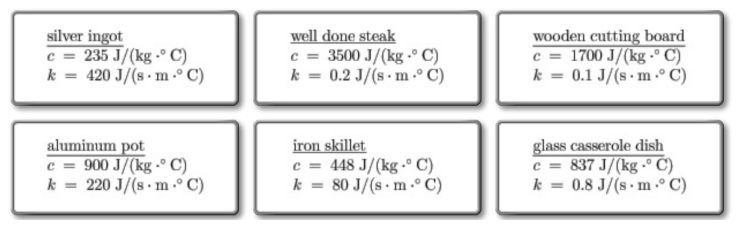# Problem: Six objects are placed in a 500°F (260°C) oven and allowed to reach thermal equilibrium. Each object has a mass of 1.0 kg. The specific heat and thermal conductivity of each substance are denoted by c and k.Each of the objects is immediately dunked in an identical tub of cold water. The tubs are quickly sealed and insulated. Rank the objects on the basis of their temperature on reaching equilibrium with the water.Rank from largest to smallest. To rank items as equivalent, overlap them.

###### FREE Expert Solution

The heat energy absorbed/emitted by an object is given by:

$\overline{){\mathbf{Q}}{\mathbf{=}}{\mathbf{m}}{\mathbf{C}}{\mathbf{∆}}{\mathbf{T}}}$

The quantity of heat stored by each object is directly proportional to its specific heat.

82% (11 ratings)###### Problem Details

Six objects are placed in a 500°F (260°C) oven and allowed to reach thermal equilibrium. Each object has a mass of 1.0 kg. The specific heat and thermal conductivity of each substance are denoted by c and k.

Each of the objects is immediately dunked in an identical tub of cold water. The tubs are quickly sealed and insulated. Rank the objects on the basis of their temperature on reaching equilibrium with the water.Rank from largest to smallest. To rank items as equivalent, overlap them.